Tamilnadu State Board New Syllabus Samacheer Kalvi 12th Maths Guide Pdf Chapter 8 Differentials and Partial Derivatives Ex 8.8 Textbook Questions and Answers, Notes.

## Tamilnadu Samacheer Kalvi 12th Maths Solutions Chapter 8 Differentials and Partial Derivatives Ex 8.8

Choose the most suitable answer from the given four alternatives:

Question 1.
A circular template has a radius of 10 cm. The measurement of the radius has an approximate error of 0.02 an. Then the percentage error in the calculating the area of this template is
(a) 0.2 %
(b) 0.4 %
(c) 0.04 %
(d) 0.08 %
Solution:
(b) 0.4 %
Hint:
Radius of a circular plate = 10 cm
error in radius dr = 0.02
A = πr²
dA = 2πrdr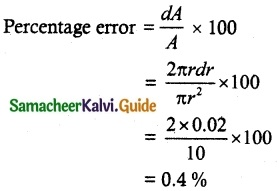Question 2.
The percentage error of the fifth root of 31 is approximately how many times the percentage error in 31?
(a) $$\frac{1}{31}$$
(b) $$\frac{1}{5}$$
(c) 5
(d) 31
Solution:
(b) $$\frac{1}{5}$$
Hint:
(i.e.) the percentage error in the nth root of a number is approximately $$\frac{1}{n}$$ times the percentage error in the number.

Question 3.
If u (x, y) = ex2 + y2 then $$\frac{\partial u}{\partial x}$$ is equal to
(a) ex2 + y2
(b) 2xu
(c) x²u
(d) y²u
Solution:
(b) 2xu
Hint:
u = ex2 + y2
$$\frac{\partial u}{\partial x}$$ = ex2 + y2 (2x) = 2xuQuestion 4.
If v (x, y) = log (ex + ey), then $$\frac{\partial v}{\partial x}$$ + $$\frac{\partial u}{\partial y}$$ is equal to
(a) (ex + ey)
(b) $$\frac{1}{e^x+e^y}$$
(c) 2
(d) 1
Solution:
(d) 1
Hint:
v = log (ex + ey)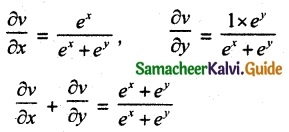= 1

Question 5.
If w (x, y) = xy, x > 0, then $$\frac{\partial w}{\partial x}$$ is equal to
(a) xy log x
(b) y log x
(c) y xy-1
(d) x log y
Solution:
(c) y xy-1
Hint:
w(x, y) = xy
$$\frac{\partial w}{\partial x}$$ = y xy-1Question 6.
If f (x, y) = exy, then $$\frac{\partial^2 f}{\partial x \partial y}$$ is equal to
(a) xy exy
(b) (1 + xy)exy
(c) (1 + y) exy
(d) (1 + x)exy
Solution:
(b) (1 + xy)exy
Hint:
f(x, y) = exy
$$\frac{\partial f}{\partial y}$$ = x exy (x) = xexy
$$\frac{\partial^2 f}{\partial x \partial y}$$ = x exy (y) + exy = exy(1 + xy)

Question 7.
If we measure the side of a cube to be 4 cm with an error of 0.1 cm, then the error in our calculation of the volume is
(a) 0.4 cu.cm
(b) 0.45 cu.cm
(c) 2 cu.cm
(d) 4.8 cu.cm
Solution:
(d) 4.8 cu.cm
Hint:
Side of the cube = 4 cm
Volume V = x³
dV = 3x²dx
= 3 × 16 × 0.1
= 4.8 cu. cmQuestion 8.
The change in the surface area S = 6x² of a cube when the edge length varies from x0 to x0 + dx is
(a) 12 x0 + dx
(b) 12 x0 dx
(c) 6 x0 dx
(d) 6 x0 + dx
Solution:
(b) 12 x0 dx
Hint:
Surface Area S = 6x²
dS = 12 × dx
dS = 12 x0 dx

Question 9.
The approximate change in volume V of a cube of side x meters caused by increasing the side by 1% is
(a) 0.3 x m³
(b) 0.03 x m³
(c) 0.03 x² m³
(d) 0.03 x³ m³
Solution:
(c) 0.03 x² m³
Hint:
Volume of the cube V = x³
dV = 3x² dx
= 3x² × 0.01
= 0.03 x² m³Question 10.
If g (x, y) = 3x² – 5y + 2y², x(t) = et and y(t) = cos t then $$\frac{dg}{dt}$$ is equal to
(a) 6 e2t + 5 sin t – 4 cos t sin t
(b) 6 e2t – 5 sin t – 4 cos t sin t
(c) 3 e2tt + 5 sin t + 4 cos t sin t
(d) 3 e2t – 5 sint + 4 cos t sin t
Solution:
(a) 6 e2t + 5 sin t – 4 cos t sin t
Hint:
x = et, y = cos t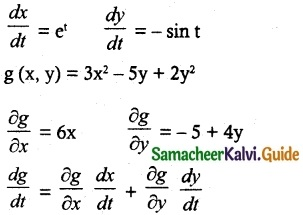= 6 x et + (-5 + 4y) – sin t
= 6 et et + 5 sin t – 4 cos t sin t
= 6e2t + 5 sin t – 4 cos t sin t

Question 11.
If f(x) = $$\frac{x}{x+1}$$, then its differential is given by
(a) –$$\frac{x}{(x+1)^2}$$ dx
(b) $$\frac{x}{(x+1)^2}$$ dx
(c) $$\frac{x}{x+1}$$ dx
(d) –$$\frac{x}{x+1}$$ dx
Solution:
(b) $$\frac{x}{(x+1)^2}$$ dx
Hint: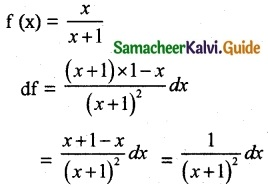Question 12.
If u (x, y) = x² + 3xy + y – 2019, then $$\frac{\partial u}{\partial x}$$| (4, -5) is equal to
(a) -4
(b) -3
(c) -7
(d) 13
Solution:
(c) -7
Hint:
u (x, y) = x² + 3xy + y – 2019
$$\frac{\partial u}{\partial x}$$ = 2x + 3y
$$\frac{\partial u}{\partial x}$$| (4, -5) = 8 – 15 = -7

Question 13.
Linear approximation for g(x) = cos x at x = $$\frac{π}{2}$$ is
(a) x + $$\frac{π}{2}$$
(b) -x + $$\frac{π}{2}$$
(c) x – $$\frac{π}{2}$$
(d) -x – $$\frac{π}{2}$$
Solution:
(b) -x + $$\frac{π}{2}$$
Hint:
linear approximation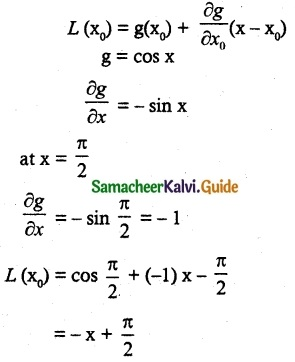Question 14.
If w (x, y, z) = x² (y – z) + y² ( z – x)+ z²(x – y) then $$\frac{\partial w}{\partial x}$$ + $$\frac{\partial w}{\partial y}$$ + $$\frac{\partial w}{\partial z}$$ is
(a) xy + yz + zx
(b) x (y + z)
(c) y (z + x)
(d) 0
Solution:
(d) 0
Hint:
w (x, y, z) = x² (y – z) + y² ( z – x)+ z²(x – y)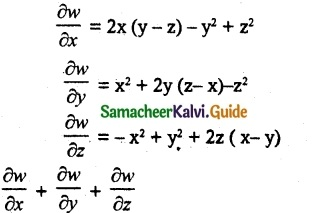= 2xy – 2xz – y² + z² + x² + 2yz – 2yx – z² – x² + y² + 2zx – 2zy
= 0

Question 15.
If f (x, y, z) = xy + yz + zx, then fx – fz is equal to
(a) z – x
(b) y – z
(c) x – z
(d) y – x
Solution:
(a) z – x
Hint:
f (x, y, z) = xy + yz + zx
$$\frac{\partial f}{\partial x}$$ = y + z
$$\frac{\partial f}{\partial z}$$ = y + x
fx – fz = y + z – y – x = z – x